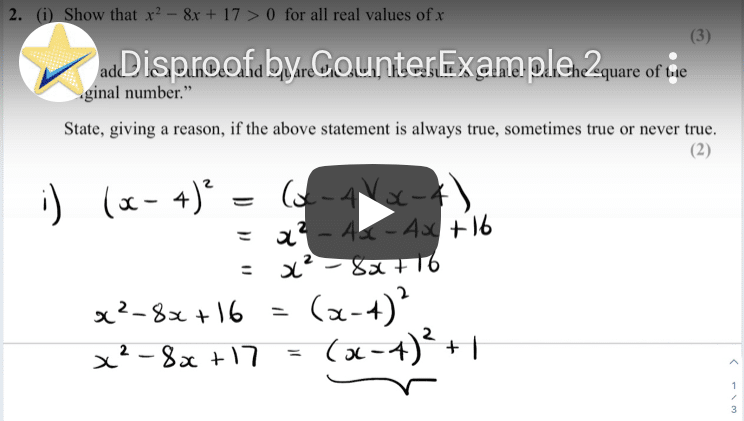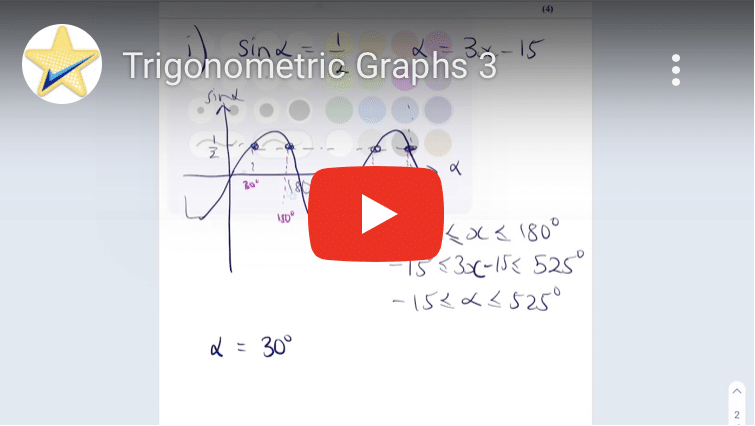# AS Pure Maths

## Proof

How to show that a specific expression is always even, regardless of the choice of integer.

Proof by Exhaustion that all square numbers are either a multiple of 4 or one more than a multiple of 4

Correcting student work and using a counterexample to disprove an algebraic statement.

Sample Assessment question requiring the use of Proof by Deduction to solve an algebraic inequality.

Proof by Deduction of an inequality using the expansion of a quadratic.

Proof by Exhaustion that all square numbers are either a multiple of 3 or one more than a multiple of 3A standard proof by deduction question mixed with a little disguised disproof by counterexample.

## Algebra & Functions

Factorising and sketching a simple cubic with a little coordinate geometry thrown in.

Sketching a cubic then performing a transformation from an unknown constant.

Sketching and finding the discriminant of a quadratic in a completing the square style question.

Sketching a quadratic against a cubic and using algebra to find their intersection points.

Solving a linear equation by simplifying a surd and rationalising a denominator first.

Completing the square with unknowns in the coefficients.

Sketching and transforming a cubic and a little differentiation.

The graph of 1/x^2 and odd and even functions.

Finding the discriminant of a quadratic with algebraic coefficients and unknown values.

Using indices rules to terms in the form of others and using them to solve hidden quadratics.

Curve Sketching and Simultaneous Equations all rolled in to an Inequalities question.

Factor Theorem and Remainder Theorem all rolled in with Simultaneous Equations and Factorising a Cubic.

A real life quadratics question rooted in the context of profit versus selling price.

Using simultaneous equations and discriminants to find an inequality in terms of unknowns.

Simplifying two surds and using the result to rationalise a denominator.

Simplifying a surd and using it to find the width of a triangle given its area and length in terms of surds.

Sketching a reciprocal function using transformations and performing various additional transformations to the original curve.

## Coordinate Geometry

Testing for perpendicularity of straight lines.

Equation of a circle by finding centre and radius and equation of a tangent.

A exam question testing combining knowledge of straight lines, GCSE Trig and problem solving.

Tricky coordinate geometry question combining straight lines, quadrilaterals and problem solving.

Past exam question on circle geometry which is heavy on trigonometry.

## Sequences & Series

A binomial expansion followed by finding missing coefficients when multiplied by a linear factor.

Binomial Expansion with an unknown coefficient combined with Simultaneous Equations.

## Trigonometry

Using a trigonometric identity to determine a cos value which is used in an application of the cosine rule.

Using trigonometric identities to transform one identity into a quadratic in cos then using this to solve a trigonometric equation.

Solution of a relatively simple trigonometric equation without using CAST or transforming a trigonometric graph.

Identifying possible values of an unknown constant by considering transformations to a sine curve.

Using the sine and cosine rule to find angles and lengths in a quadrilateral flowerbed.

Using a trigonometric identity to solve a pretty horrendous trigonometric equation.

A not-so obvious difference of squares in terms of trigonometric functions which simplifies to a single cos term.

A slightly different type of trigonometric equations question with the discussion of multiple values of an unknown.

Transformations of a sine curve that is used to represent water depth.Finding multiple solutions of a trigonometric equation without and successive transformations to a sine curve.

## Exponentials & Logs

Correcting mistakes made in a typical student solution to a logs question.

A simple example of evaluating and differentiating a basic exponential function.

Finding exact solutions to a logs equation and an exponential equation using log rules.

Finding and interpreting the values of two unknowns that determine the exponential relationship between the value of a rare painting and time.

Using simultaneous equations to find (and then interpreting) the unknown constants that determines the exponential growth in the value of a vintage car.

A demonstration of how to find the equation of a tangent to an exponential curve.

## Differentiation

The differentiation of a polynomial that requires reformatting before differentiating.

Using derivatives to show that a function is always decreasing.

Using differentiation to locate and classify the minimum of the cost of a journey.

Finding the derivative of a polynomial function and using it to find the equation of a normal at a given point on the function’s curve.

A simple example of evaluating and differentiating a basic exponential function.

Differentiation of the cosine function from first principles using a trigonometric identity and two given basic limits.

Using stationary points and the intervals for where a function is increasing or decreasing to sketch the function’s derivative.

Using stationary points to sketch a functions that is a combination of a reciprocal and a cubic function.

Using the definition of parallel lines in gradients to find unknown an polynomial coefficient and subsequently the equation of a tangent.

A demonstration of how to find the equation of a tangent to an exponential curve.

## Integration

Integration question that requires equating coefficients.

An integral with limits that involves an unknown constant.

Integration of a polynomial including rearranging and unknown coefficients.

Approximating the integral of a binomial to a high power using binomial expansion.

Definite Integration involving a quadratic and a straight lines hence requiring knowledge of simultaneous equations as well as how to find complex areas beneath a curve.

How to find the area sandwiched between two quadratic curves.

## Vectors

Finding the vector between two points and its magnitude.

Navigating along various known vectors to obtain unknown vectors and showing that three points are colinear.

Using parallel lines to find the value of an unknown constant.

Using magnitude to find the value of an unknown in a vector then using it to find a unit vector with calculations heavily based on surds.

Finding expressions for various vectors running along the perimeter of a trapezium.

A complicated vector arithmetic question involving the position vector of a point with unknown location.

Using points and position vectors to find a new position vector and using magnitude to find the value of an unknown constant.

# AS Mechanics

## SUVAT Equations

How to select which SUVAT equation to use to calculate time for a ball to be thrown upwards before reaching the ground.

Using SUVAT Equations and a velocity-time graph question.

## Newton's Laws of Motion

A very basic introduction to balanced and unbalanced forces and Newton’s First Law of Motion.

Using Newtons 2nd Law to find the mass of a particle accelerating under gravity in two opposing directions.

Using Newton’s Laws to find the thrust between two connected particles acted on by an external force as well as gravity